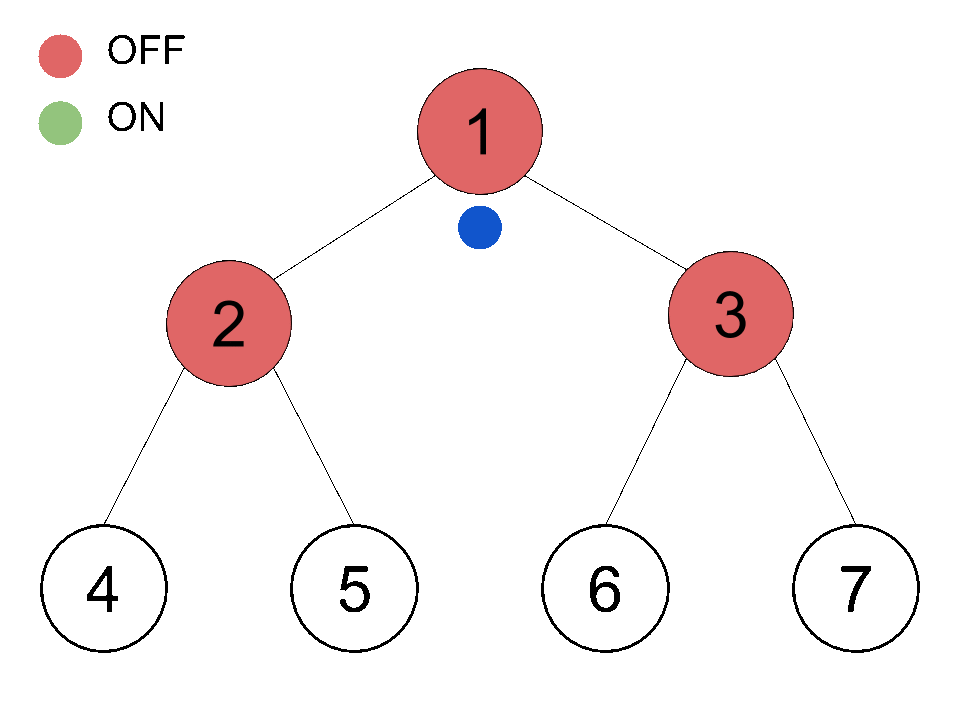Computer Science

# Binary Search Trees: Level 3 challengesThe binary search tree above has a height of 2 and has 4 leaves. You are going to pass down a ball from the root (the topmost node). Every node other than the leaves has a switch. If a node is switched off, the ball will pass to its left child; if a node is switched on, the ball will pass to its right child. After passing the ball, the switch will toggle (if it's on it becomes off and vice versa). Initially, every node is switched off.

Chris built a similar tree of height 3 with the same indexing. Which leaf will contain the $5^\text{th}$ ball?

Note: the indexes of the leaves should be in the range $[8,16)$.

###### Do you find this too simple? Try the Medium version of this problem.

Given an array and an element $x$, the floor of an element $x$ is defined as the greatest element present in the array which is less than or equal to $x$.

What is the worst case complexity of the most efficient algorithm for finding a floor of an element $x$ in a sorted array?

Details and Assumptions:

• If the array is $[3, 8, 15, 19, 23]$ and $x=20$, then the output will be $19$.

• $x$ can't be less than the minimum element in the list.

What data structure is required for storing a set of integers such that deletion of the smallest element and insertion of an element not already present in the set can each be done in $O(\log n)$ time?

×

Problem Loading...

Note Loading...

Set Loading...